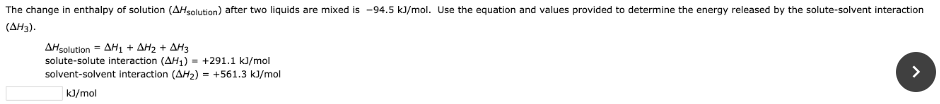# The change in enthalpy of solution (ΔHsolution) after two liquids are mixed is -94.5 kJ/mol. Use the equation and values provided to determine the energy released by the solute-solvent interaction (ΔH3). ΔHsolution = ΔH1 + ΔH2 + ΔH3 solute-solvent interaction (ΔH1) = +291.1 kJ/mol solvent-solvent interaction (ΔH2) = +561.3 kJ/mol